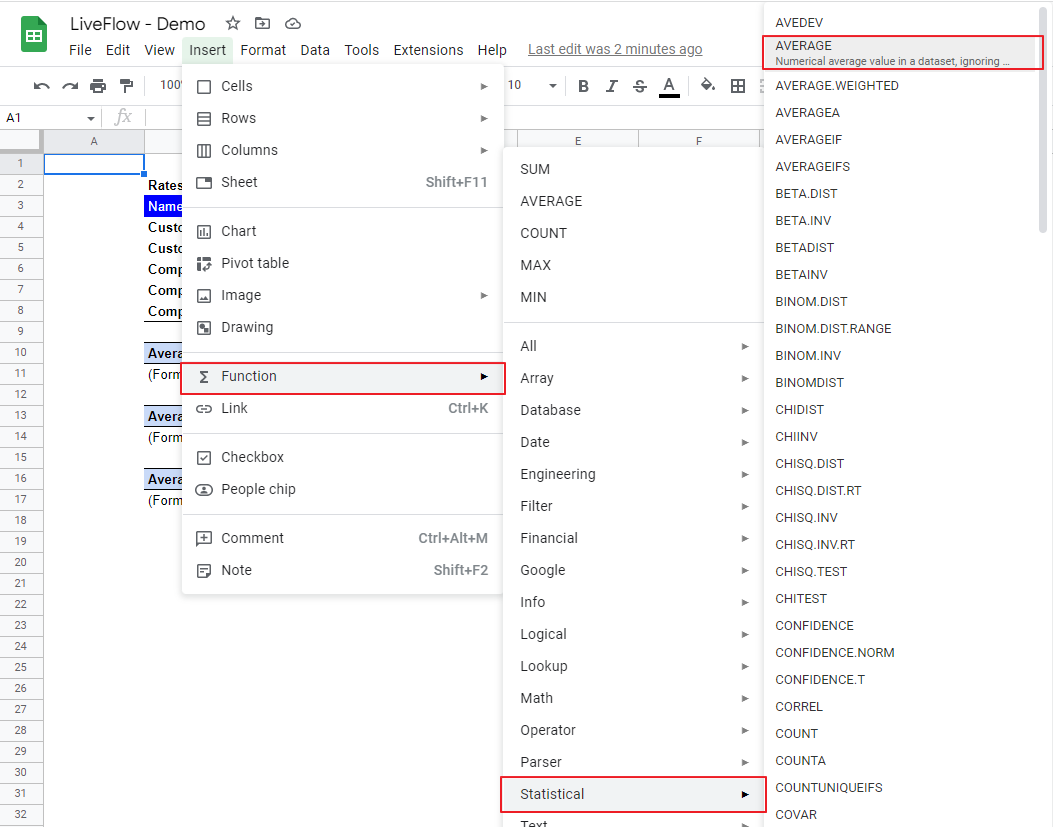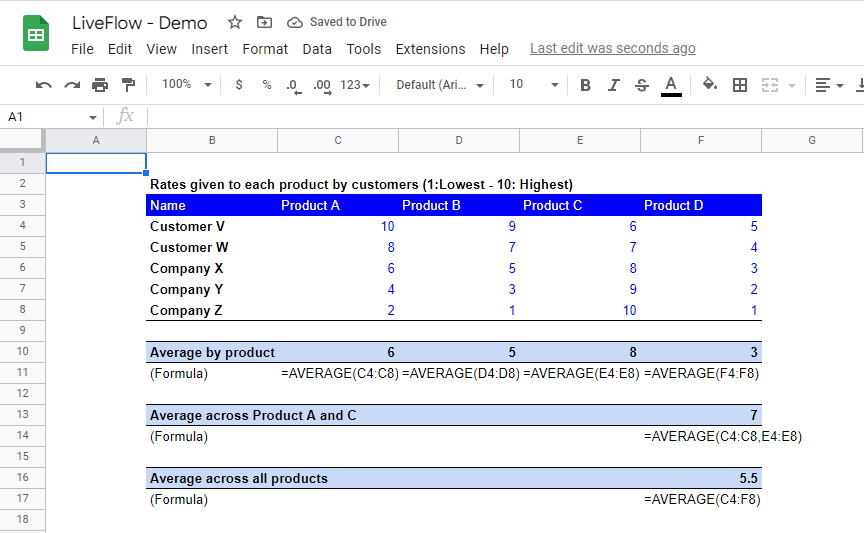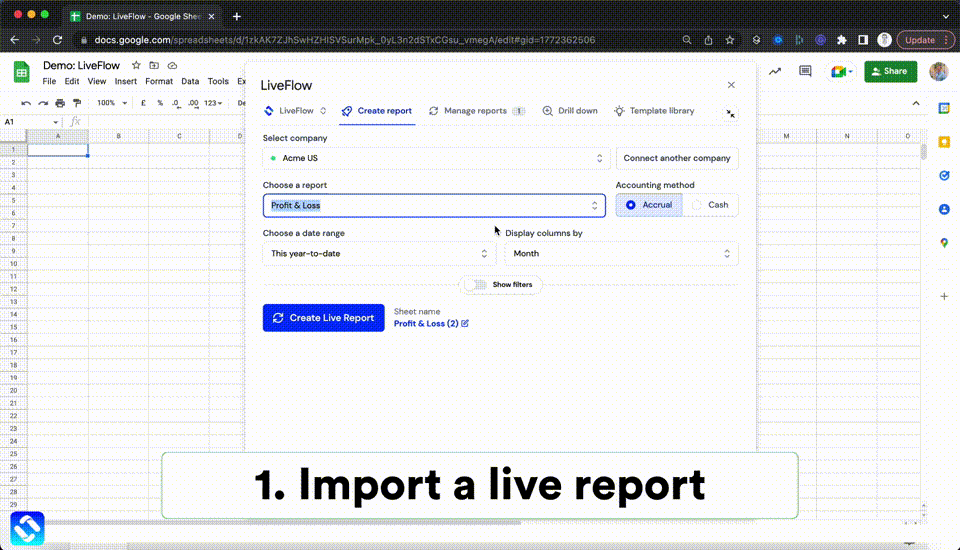# How to Use AVERAGE Formula in Google Sheets

In this article, you will learn how to find the mean number in a list of numbers. This function is beneficial when you calculate the average number from a long list of numbers. The mean (or average) number of a set of numbers is the middle value, computed by dividing the sum of all the values in the set by the number of values included in the set.

## How to Use Average formula in Google Sheets

1. Type “=AVERAGE(” or go to “Insert” in the menu bar ➝ “Function” ➝ ”Statistical” ➝ ”AVERAGE”
2. Select a set of data in which you want to find the median. If you're going to incorporate non-adjacent areas, you can include them by punctuating them with a comma(s).
3. Press the “Enter” key.How to insert the AVERAGE function from the menu bar

The generic formula is as follows:

``=AVERAGE(value1, [value2, …])``

Value 1: A set of data based on which you want to figure out the mean.

Let’s see some examples. Imagine you review five customers’ rates given to four types of products:How to use the AVERAGE function in Google Sheets with some examples

(i) Average by product

This is very simple. You can just select a part of the column for each product. For example, the AVERAGE formula in cell C10 (for Product A) contains a range of C4:C8, precisely 10, 8, 6, 4, and 2, and you get 6 as a computed result.

(ii) Average across Product A and C

This example might be a little tricky. As mentioned above, if you want to select non-adjacent fields as a data set, you can use a comma(s). Select a part of the column for Product A (C4:C8), add a comma, and select a part of the column for Product C (E4:E8). The total value of the specified range is 70 (=10+8+6+4+2+6+7+8+9+10), and the number of values in the chosen field is 10. So, the result is 7, from 70 divided by 10.

As you can see in the screenshot, you can select a table as a set of data which include twenty figures for all product. The total value in the range is 110, the average is 5.5, which comes from the division of 110 over 20.

## How do you find the average of multiple cells in Google Sheets?

You can use the AVERAGE function equipped in Google Sheets. As you can see in the description above, you can incorporate a set of cells or multiple non-adjacent ranges of cells with commas in the formula.

## Is there an AVERAGEIF function in Google Sheets?

Yes, there is. Check this article to learn about the AVERAGEIF formula in Google Sheets. There is also a function called MEDIAN, which allows you to find the median number in a set of data as you do with the AVERAGE formula. Check How to Use MEDIAN function in Google Sheets.

## Analyze your live financial data in a snap

Are you learning this formula to process financial data, build a financial model, or conduct financial analysis? If so, LiveFlow may help you to automate manual workflows and keep numbers updated in real time. For instance, you can easily compare your Budget versus Actual without any manual work. Furthermore, you can access more than fifty templates here, from the simple Income Statement and Operational KPI templates to Multi-Currency Consolidated Income Statement, Balance Sheet, and Cash Flow templates. You can also customize these templates as you want without breaking the automated data inflow.Automate any custom financial dashboard in Google Sheets with LiveFlow

Learn how to do this step-by-step in the video below 👇

## Do you need personal help?

Our team of real people are here to help you any time between 9am and 10pm GMT.Email us at: founders@liveflow.ioCall us at +1 (415) 650-1711